•••# Biogas generator sets from 60 kWe to 3770 kWe

## > Biogas generator sets from 60 to 530 kWe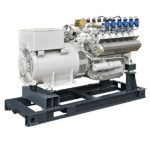Main characteristics
• Electrical rating: 63 kWe
• Heat Recovery: 86 kW
• Energy consumption: 179 kW COW
• Electrical efficiency: 35,2%
• Cogeneration efficiency: 83,2%Main characteristics
• Electrical rating: 104 kWe
• Heat Recovery: 125 kW
• Energy consumption: 274 kW COW
• Electrical efficiency: 38%
• Cogeneration efficiency: 83,6%Main characteristics
• Electrical rating: 123 kWe
• Heat Recovery: 168 kW
• Energy consumption: 343 kW COW
• Electrical efficiency: 35,9%
• Cogeneration efficiency: 84,8%Main characteristics
• Electrical rating: 191 kWe
• Heat Recovery: 243 kW
• Energy consumption: 520 kW COW
• Electrical efficiency: 36,7%
• Cogeneration efficiency: 83,5%Main characteristics
• Electrical rating: 209 kWe
• Heat Recovery: 213 kW
• Energy consumption: 521 kW COW
• Electrical efficiency: 40,1%
• Cogeneration efficiency: 81%Main characteristics
• Electrical rating: 253 kWe
• Heat Recovery: 299 kW
• Energy consumption: 657 kW COW
• Electrical efficiency: 38,5%
• Cogeneration efficiency: 84%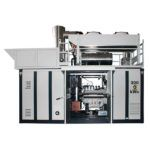Main characteristics
• Electrical rating: 366 kWe
• Heat Recovery: 435 kW
• Energy consumption: 946 kW COW
• Electrical efficiency: 38,7%
• Cogeneration efficiency: 84,7%Main characteristics
• Electrical rating: 404 kWe
• Heat Recovery: 492 kW
• Energy consumption: 1050 kW COW
• Electrical efficiency: 38,5%
• Cogeneration efficiency: 85,3%Main characteristics
• Electrical rating: 530 kWe
• Heat Recovery: 599 kW
• Energy consumption: 1341 kW COW
• Electrical efficiency: 39,5%
• Cogeneration efficiency: 84,2%

## > Biogas generator sets from 400 to 3770 kWe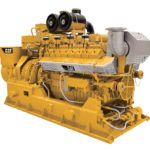Main characteristics
• Electrical rating: 400 kWe
• Heat Recovery: 375 kW
• Energy consumption: 935 kW COW
• Electrical efficiency: 42,8%
• Cogeneration efficiency: 82,9%Main characteristics
• Electrical rating: 600 kWe
• Heat Recovery: 566 kW
• Energy consumption: 1405 kW COW
• Electrical efficiency: 42,7%
• Cogeneration efficiency: 83%Main characteristics
• Electrical rating: 800 kWe
• Heat Recovery: 752 kW
• Energy consumption: 1871 kW COW
• Electrical efficiency: 42,8%
• Cogeneration efficiency: 83%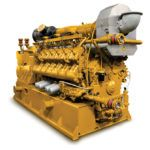Main characteristics
• Electrical rating: 1000 kWe
• Heat Recovery: 1191 kW
• Energy consumption: 2852 kW COW
• Electrical efficiency: 42,1%
• Cogeneration efficiency: 83,8%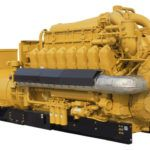Main characteristics
• Electrical rating: 1091 kWe
• Heat Recovery: 1202 kW
• Energy consumption: 3001 kW COW
• Electrical efficiency: 36,4%
• Cogeneration efficiency: 76,4%Main characteristics
• Electrical rating: 1200 kWe
• Heat Recovery: 1191 kW
• Energy consumption: 2852 kW COW
• Electrical efficiency: 42,1%
• Cogeneration efficiency: 83,8%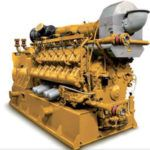Main characteristics
• Electrical rating: 1560 kWe
• Heat Recovery: 1567 kW
• Energy consumption: 3739 kW COW
• Electrical efficiency: 41,7%
• Cogeneration efficiency: 83,6%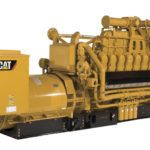Main characteristics
• Electrical rating: 1600 kWe
• Heat Recovery: 1667 kW
• Energy consumption: 4003 kW COW
• Electrical efficiency: 40%
• Cogeneration efficiency: 81,6%Main characteristics
• Electrical rating: 1993 kWe
• Heat Recovery: 1986 kW
• Energy consumption: 4788 kW COW
• Electrical efficiency: 41,6%
• Cogeneration efficiency: 83,1%Main characteristics
• Electrical rating: 2000 kWe
• Heat Recovery: 2008 kW
• Energy consumption: 4762 kW COW
• Electrical efficiency: 42%
• Cogeneration efficiency: 84,2%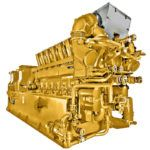Main characteristics
• Electrical rating: 2830 kWe
• Heat Recovery: 2920 kW
• Energy consumption: 6690 kW COW
• Electrical efficiency: 42,3%
• Cogeneration efficiency: 85,9%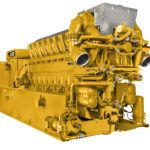Main characteristics
• Electrical rating: 3770 kWe
• Heat Recovery: 3856 kW
• Energy consumption: 8870 kW COW
• Electrical efficiency: 42,5%
• Cogeneration efficiency: 86%

top